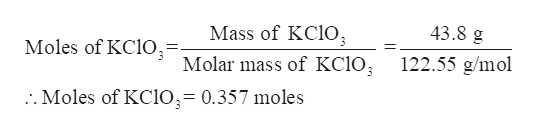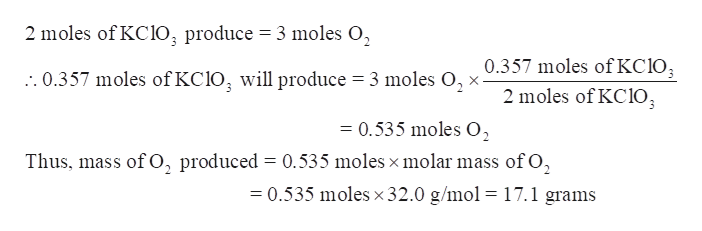# Small quantities of oxygen can be prepared in the laboratory by heating potassium chlorate, KClO3(s).KClO3(s).The equation for the reaction is 2KClO3⟶2KCl+3O22KClO3⟶2KCl+3O2 Calculate how many grams of O2(g)O2(g) can be produced from heating 43.8 grams43.8 grams of KClO3(s).KClO3(s).STRATEGYConvert the mass of KClO3KClO3 to moles.Convert the number of moles of KClO3KClO3 to the number of moles of O2.O2.Convert the number of moles of O2O2 to grams.Step 1: 43.8 g43.8 g of KClO3KClO3 is equal to 0.3570.357 mol KClO3.KClO3.Step 2: 0.357 mol0.357 mol KClO3KClO3 corresponds to 0.536 mol 0.536 mol O2.O2.Step 3: Convert 0.536 mol0.536 mol O2O2 to grams.

Question
44 views

Small quantities of oxygen can be prepared in the laboratory by heating potassium chlorate, KClO3(s).KClO3(s).

The equation for the reaction is

2KClO3⟶2KCl+3O22KClO3⟶2KCl+3O2

Calculate how many grams of O2(g)O2(g) can be produced from heating 43.8 grams43.8 grams of KClO3(s).KClO3(s).

STRATEGY

1. Convert the mass of KClO3KClO3 to moles.
2. Convert the number of moles of KClO3KClO3 to the number of moles of O2.O2.
3. Convert the number of moles of O2O2 to grams.

Step 1: 43.8 g43.8 g of KClO3KClO3 is equal to 0.3570.357 mol KClO3.KClO3.

Step 2: 0.357 mol0.357 mol KClO3KClO3 corresponds to 0.536 mol 0.536 mol O2.O2.

Step 3: Convert 0.536 mol0.536 mol O2O2 to grams.

check_circle

Step 1

Small quantities of oxygen can be prepared in the laboratory by heating potassium chlorate, KClO3(s).

The grams of O2(g) can be produced from heating 43.8 grams KClO3(s).

Step 2

Calculate the moles of KClO3 in 43.8 grams-

Given mass of KClO3 = 43.8 g

Molar mass of KClO3 = 122.55 g/molhelp_outlineImage Transcriptionclose43.8 g Mass of KC1O Molar mass of KC1O122.55 g/mol Moles of KC1O3= :. Moles of KCIO;= 0.357 moles fullscreen
Step 3

Determine the moles and mass of O2(g) produced-

As per the balanced equation, 2 moles of KCl...help_outlineImage Transcriptionclose2 moles of KC1O, produce 3 moles O 0.357 moles of KC103 2 moles of KCIO :. 0.357 moles of KC10, will produce = 3 moles O =0.535 moles O Thus, mass of , produced 0.535 moles x molar mass of O, =0.535 moles x 32.0 g/mol = 17.1 grams fullscreen

### Want to see the full answer?

See Solution

#### Want to see this answer and more?

Solutions are written by subject experts who are available 24/7. Questions are typically answered within 1 hour.*

See Solution
*Response times may vary by subject and question.
Tagged in

### General Chemistry